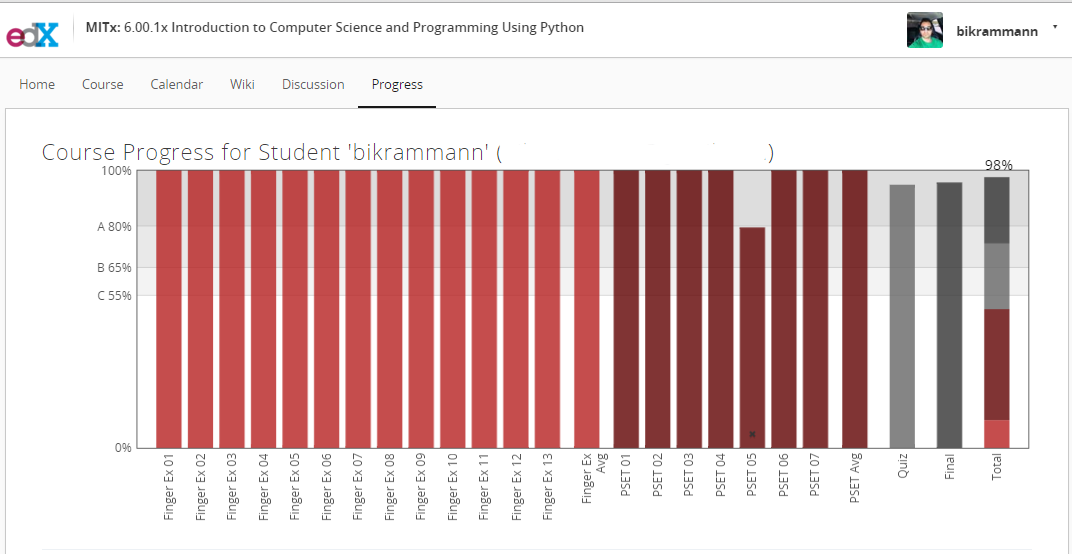MITx: 6.00.1x Introduction to Computer Science and Programming Using PythonMITx: 6.00.1x Introduction to Computer Science and Programming Using Python

Just got done taking MIT’s Python Course “Introduction to Computer Science and Programming – 6.00.1x “. This course is taught by MIT Professor Eric Grimson via edX.org and covers the same material which is taught in class at MIT. Edx version of MIT course is broken in two parts:

PART 1:
MITx: 6.00.1x Introduction to Computer Science and Programming Using Python
https://www.edx.org/course/introduction-computer-science-mitx-6-00-1x-6

PART 2:
MITx: 6.00.2x Introduction to Computational Thinking and Data Science
https://www.edx.org/course/introduction-computational-thinking-data-mitx-6-00-2x-3

MITx: 6.00.1x – Review

• Length – 9 weeks
• Effort – 15 hours/week
• Institution – MITx

This course has 13 finger exercises, 7 problem sets, 1 mid term quiz and 1 final exam.

• Finger Exercises – 10%
• Problem Sets – 40%
• Mid Term Quiz – 25%
• Final Exam – 25%

Topics you will learn about:

• Lecture 1 – Introduction to Computation
• Lecture 2 – Core Elements of Programs
• Lecture 3 – Simple Algorithms
• Lecture 4 – Functions
• Lecture 5 – Recursion
• Lecture 6 – Objects
• Lecture 7 – Debugging
• Lecture 8 – Assertions and Exceptions
• Lecture 9 – Efficiency and Orders of Growth
• Lecture 10 – Memory and Search
• Lecture 11 – Classes
• Lecture 12 – Object Oriented Programming
• Lecture 13 – Trees

This was one of the best programming course that I have taken so far. Not only you will learn how to program but you will also get good overview of computer science concepts in general such as Algorithms, Recursion, Debugging, Assertions and Exceptions, Efficiency and Orders of Growth. This course will give you enough confidence to move on to more advance courses like how compilers work, operating systems, data structures & algorithms etc. Learning the basics and syntax of any programming language is important but in order to be a better programmer you have to understand these core concepts.